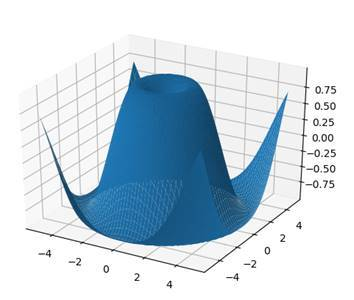# Data Science Posts and Resources

Articles on Data Science

## Python - Matplotlib- Plot Types

Matplotlib- Plot Types

Laxmi K Soni## MATPLOTLIB PLOT TYPES

Matplotlib offers a huge arsenal of different plot types. Here we are going to take a look at these.

#### HISTOGRAMS

Let’s start out with some statistics here. So-called histograms represent the distribution of numerical values. For example, we could graph the distribution of heights amongst students in a class.

mu, sigma = 172 , 4
x = mu + sigma * np.random.randn( 10000 ) 

We start by defining a mean value mu (average height) and a standard deviation sigma . To create our x-values, we use our mu and sigma combined with 10000 randomly generated values. Notice that we are using the randn function here. This function generates values for a standard normal distribution , which means that we will get a bell curve of values.

plt.hist(x, 100 , density = True , facecolor = 'blue' ) 

Then we use the hist function, in order to plot our histogram. The second parameter states how many values we want to plot. Also, we want our values to be normed. So we set the parameter density to True . This means that our y-values will sum up to one and we can view them as percentages. Last but not least, we set the color to blue.

Now, when we show this plot, we will realize that it is a bit confusing. So we are going to add some labeling here.

plt.xlabel( 'Height' )
plt.ylabel( 'Probability' )
plt.title( 'Height of Students' )
plt.text( 160 , 0.125 , 'µ = 172, σ = 4' )
plt.axis([ 155 , 190 , 0 , 0.15 ])
plt.grid( True )

First we label the two axes. The x-values represent the height of the students, whereas the y-values represent the probability that a randomly picked student has the respective height. Besides the title, we also add some text to our graph. We place it at the x-value 160 and the y-value of 0.125. The text just states the values for µ (mu) and σ (sigma).

Last but not least, we set the ranges for the two axes. Our x-values range from 155 to 190 and our y-values from 0 to 0.15. Also, the grid is turned on. This is what our graph looks like at the end: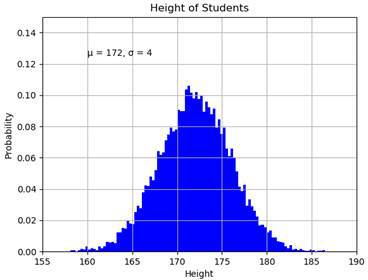We can see the Gaussian bell curve which is typical for the standard normal distribution.

#### BAR CHART

For visualizing certain statistics, bar charts are oftentimes very useful, especially when it comes to categories. In our case, we are going to plot the skill levels of three different people in the IT realm.

bob = ( 90 , 67 , 87 , 76 )
charles = ( 80 , 80 , 47 , 66 )
daniel = ( 40 , 95 , 76 , 89 )
skills = ( 'Python' , 'Java' , 'Networking' , 'Machine Learning' )

Here we have the three persons Bob, Charles and Daniel . They are represented by tuples with four values that indicate their skill levels in Python programming, Java programming, networking and machine learning.

width = 0.2
index = np.arange( 4 )
plt.bar(index, bob,
width =width, label = 'Bob' )
plt.bar(index + width, charles,
width =width, label = 'Charles' )
plt.bar(index + width * 2 , daniel,width =width, label = 'Daniel' )

We then use the bar function to plot our bar chart. For this, we define an array with the indices one to four and a bar width of 0.2. For each person we plot the four respective values and label them.

plt.xticks(index + width, skills)
plt.ylim( 0 , 120 )
plt.title( 'IT Skill Levels' )
plt.ylabel( 'Skill Level' )
plt.xlabel( 'IT Skill' )
plt.legend()

Then we label the x-ticks with the method xticks and set the limit of the y-axis to 120 to free up some space for our legend. After that we set a title and label the axes. The result looks like this:We can now see who is the most skilled in each category. Of course we could also change the graph so that we have the persons on the x-axis with the skill-colors in the legend.

#### PIE CHART

Pie charts are used to display proportions of numbers. For example, we could graph how many percent of the students have which nationality.

labels = ( 'American' , 'German' , 'French' , 'Other' )
values = ( 47 , 23 , 20 , 10 ) 

We have one tuple with our four nationalities. They will be our labels. And we also have one tuple with the percentages.

plt.pie(values, labels =labels,
autopct = '%.2f%%' , shadow = True )
plt.title( 'Student Nationalities' )
plt.show()

Now we just need to use the pie function, to draw our chart. We pass our values and our labels. Then we set the autopct parameter to our desired percentage format. Also, we turn on the shadow of the chart and set a title. And this is what we end up with: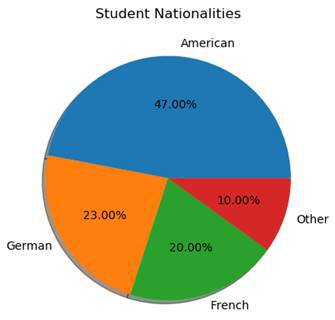As you can see, this chart is perfect for visualizing percentages.

#### SCATTER PLOTS

So-called scatter plots are used to represent two-dimensional data using dots.

x = np.random.rand( 50 )
y = np.random.rand( 50 )
plt.scatter(x,y)
plt.show()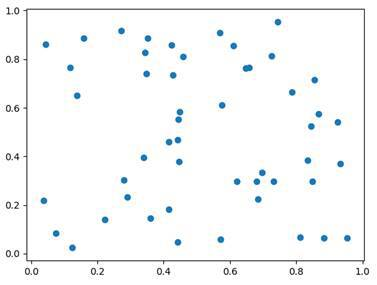Here we just generate 50 random x-values and 50 random y-values. By using the scatter function, we can then plot them.

#### BOXPLOT

Boxplot diagrams are used, in order to split data into quartiles . We do that to get information about the distribution of our values. The question we want to answer is: How widely spread is the data in each of the quartiles.

mu, sigma = 172 , 4
values = np.random.normal(mu,sigma, 200 )
plt.boxplot(values)
plt.title( 'Student's Height' )
plt.ylabel( 'Height' )
plt.show()

In this example, we again create a normal distribution of the heights of our students. Our mean value is 172, our standard deviation 4 and we generate 200 values. Then we plot our boxplot diagram.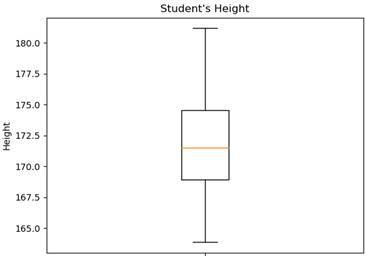Here we see the result. Notice that a boxplot doesn’t give information about the frequency of the individual values. It only gives information about the spread of the values in the individual quartiles. Every quartile has 25% of the values but some have a very small spread whereas others have quite a large one.

#### 3D PLOTS

Now last but not least, let’s take a look at 3D-plotting. For this, we will need to import another plotting module. It is called mpl_toolkits and it is part of the Matplotlib stack.

from mpl_toolkits import mplot3d

Specifically, we import the module mplot3d from this library. Then, we can use 3d as a parameter when defining our axes.

ax = plt.axes( projection = '3d' )
plt.show()

We can only use this parameter, when mplot3d is imported. Now, our plot looks like this: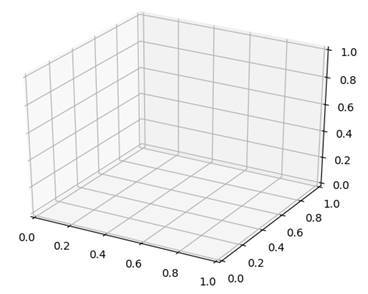Since we are now plotting in three dimensions, we will also need to define three axes.

z = np.linspace( 0 , 20 , 100 )
x = np.sin(z)
y = np.cos(z)
ax = plt.axes( projection = '3d' )
ax.plot3D(x,y,z)
plt.show()

In this case, we are taking the z-axis as the input. The z-axis is the one which goes upwards. We define the x-axis and the y-axis to be a sine and cosine function. Then, we use the function plot3D to plot our function. We end up with this: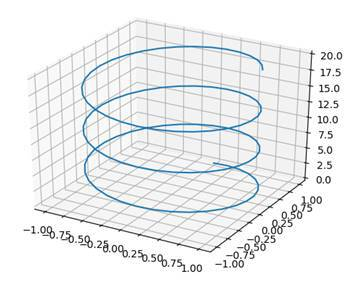#### SURFACE PLOTS

Now in order to plot a function with a surface, we need to calculate every point on it. This is impossible, which is why we are just going to calculate enough to estimate the graph. In this case, x and y will be the input and the z-function will be the 3D-result which is composed of them.

ax = plt.axes( projection = '3d' )
def z_function(x, y):
return np.sin(np.sqrt(x ** 2 + y ** 2 ))
x = np.linspace(- 5 , 5 , 50 )
y = np.linspace(- 5 , 5 , 50 )

We start by defining a z_function which is a combination of sine, square root and squaring the input. Our inputs are just 50 numbers from -5 to 5.

X, Y = np.meshgrid(x,y)
Z = z_function(X,Y)
ax.plot_surface(X,Y,Z)
plt.show()

Then we define new variables for x and y (we are using capitals this time). What we do is converting the x- and y-vectors into matrices using the meshgrid function. Finally, we use the z_function to calculate our z-values and then we plot our surface by using the method plot_surface